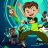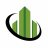# Volume Reversal Model for ThinkorSwim#### BenTen

Staff
VIP
Here's a "nice model of volume" with reversal signals in arrows and current trend painted as color candles.

Brought to you by @diazlaz### thinkScript Code

Code:
``````#Volume Game (vg) by RafaelZioni
#
# 2019.11.23 1.0 @diazlaz - Original Port

#declare zerobase;

input RSIlength = 6; #RSI Period Length
input RSIoverSold = 50;
input RSIoverBought = 50;
input price = close;

#///////////// RSI
def vrsi = RSI(RSIlength, RSIoverBought, RSIoverSold, price);

#///////////// Bollinger Bands
input BBlength = 200; #Bollinger Period Length
input BBmult = 2; #Bollinger Bands Standard Deviation
def BBbasis = Average(price, BBlength);
def BBdev = BBmult * StDev(price, BBlength);
def BBupper = BBbasis + BBdev;
def BBlower = BBbasis - BBdev;
def source = close;
def buyEntry = Crosses(source, BBlower, CrossingDirection.ABOVE);
def sellEntry = Crosses(source, BBupper, CrossingDirection.BELOW);
input window1 = 8; #lookback window 1
input window2 = 21; #lookback window 2

def top1 = If(high >= Highest(high, window1), high, 0);
def bot1 = If(low <= Lowest(low, window1), low, 0);
def top2 = If(high >= Highest(high, window2), high, 0);
def bot2 = If(low <= Lowest(low, window2), low, 0);

input length = 9; #length
def avrg = Average(volume,length);

def vold1 = volume >= avrg * 1.5 and close < open;
def vold2 = volume >= avrg * 0.5 and volume <= avrg * 1.5 and close < open;
def vold3 = volume < avrg * 0.5 and close < open and volume >= avrg * 1.4;

def volu1 = volume >= avrg * 1.5 and close > open;
def volu2 = volume >= avrg * 0.6 and close > open;
def volu3 = volume < avrg * 0.5 and close > open;

def src = close;

#net volume of positive and negative volume
def nv = if (src - src) > 0 then volume else
if (src - src) < 0 then -volume else 0 * volume;

def cnv = TotalSum(nv); #cumulative net volume
def cnv_tb = cnv - Average(cnv,15); #normalized 15 bars moving average
input window_len = 28; #Window length
input v_len = 14; #V smooth length

def v =  cnv - Average(cnv,15);
def smooth = Average(v, v_len);
def v_spread = stdev(v - smooth, window_len);

def down = out < close and Crosses(out, top1, CrossingDirection.BELOW) and vold1;
def up1 = out > close and volu2 and vrsi < RSIoverSold;
def down1= out < close and vold1;
def down2= out < close;

def up = out > close and Crosses(out, bot2, CrossingDirection.ABOVE);

plot pDown = if down then high else Double.NaN;
pDown.SetPaintingStrategy(PaintingStrategy.BOOLEAN_ARROW_DOWN);
pDown.SetLineWeight(1);
pDown.AssignValueColor(COLOR.MAGENTA);

#plot pDown1 = if down2 then high else Double.NaN;
#pDown1.SetPaintingStrategy(PaintingStrategy.BOOLEAN_ARROW_DOWN);
#pDown1.SetLineWeight(1);
#pDown1.AssignValueColor(COLOR.ORANGE);

plot pUp = if up then low else Double.NaN;
pUp.SetPaintingStrategy(PaintingStrategy.BOOLEAN_ARROW_UP);
pUp.SetLineWeight(1);
pUp.AssignValueColor(COLOR.CYAN);

#plot pUp1 = if up1 then low else Double.NaN;
#pUp1.SetPaintingStrategy(PaintingStrategy.BOOLEAN_ARROW_UP);
#pUp1.SetLineWeight(1);
#pUp1.AssignValueColor(COLOR.BLUE);

assignPriceColor(if down2 then color.red else if Up1 then color.green else color.white);``````

•#### zeek

##### Active member
2019 Donor
Really nice script! Hope to see more like this one added to Warehouse which uses volume to analyse for reversals.
Thanks@BenTen

•BenTen#### BenTen

Staff
VIP

Code:
``````# Alerts

•zeek#### hectorgasm

##### New member
@BenTen does it repaint?#### zeek

##### Active member
2019 Donor
No, it does not repaint.Volume Based Reversal Candles for ThinkorSwim Custom 0Volume Spread Analysis (VSA) Reversal Indicator for ThinkorSwim Indicators 22Volume Oscillator for ThinkorSwim Indicators 2